# Distance Formula

## Coordinate Geometry of Class 10

The distance between two points P(x1, y1) and Q(x2, y2) is given by the formula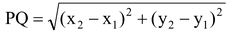.

Proof: Draw PR and QS perpendicular to the x-axis. A perpendicular from the point P on QS is drawn to meet it at the point T.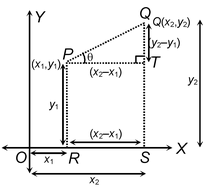Then OR = x1, OS = x2, So, RS = x2 – x1 = PT.

Also SQ = y2, ST = PR = y1. So, QT = y2 – y1.

Now applying the Pythagoras theorem in ΔPTQ,

we get PQ2 = PT2 + QT2

= (x2 – x1)2 + (y2 – y1)2.

ThereforeSince distance is always non-negative, we take only the positive square root. So the distance between the points P(x1, y1) and Q(x2, y2) iswhich is called the distance formula.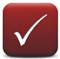In particular, the distance of a point P(x, y) from the origin O(0, 0) is given by OP = √x2 + y2.

### SOME USEFUL POINTS:

question 1. In questions relating to geometrical figures, take the given vertices in the given order and proceed as indicated.

(i) For an isosceles triangle - We have to prove that at least two sides are equal.

(ii) For an equilateral triangle - We have to prove that three sides are equal.

(iii) For a right -angled triangle - We have to prove that the sum of the squares of two sides is equal to the square of the third side.

(iv) For a square - We have to prove that the four sides are equal, two diagonals are equal.

(v) For a rhombus - We have to prove that four sides are equal (and there is no need to establish that two diagonals are unequal as the square is also a rhombus).

(vi) For a rectangle - We have to prove that the opposite sides are equal and two diagonals are equal.

(vii) For a Parallelogram - We have to prove that the opposite sides are equal (and there is no need to establish that two diagonals are unequal the rectangle is also a parallelogram).

2. For three points to be collinear - We have to prove that the sum of the distances between two pairs of points is equal to the third pair of points.

question 1. Find the distance between the points (8 , -2) and (3, -6).

question Let the points (8, -2) and (3, -6) be denoted by P and Q, respectively.

Then, by distance formula, we obtain the distance PQ asquestion 2. Find the distance between the points P(–6, 7) and Q(–1, –5).

question Here, x1 = –6, y1 = 7 and x2 = –1, y2 = –5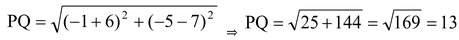question 3. Show that the points (1, –1), (5, 2) and (9, 5) are collinear.

question Let A(1, – 1), B(5, 2) and (9, 5) be the given points. Then we have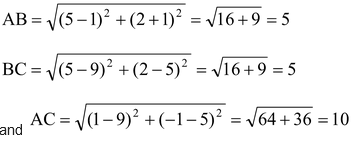Clearly, AC = AB + BC.

Hence, A, B, C are collinear points.

question 4. Prove that the points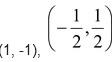and (1, 2) are the vertices of an isosceles triangle.

question Let the pointand (1, 2) be denoted by P, Q and R, respectively. Now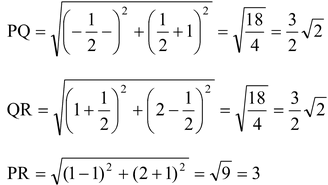From the above, we see that PQ = QR.

∴ The triangle is isosceles.

question 5. Using distance formula, show that the points (-3, 2), (1, -2) and (9, -10) are collinear.

question Let the given points (-3, 2), (1, -2) and (9, -10) be denoted by A, B and C, respectively. Points A, B and C will be collinear, if the sum of the lengths of two line-segments is equal to the third.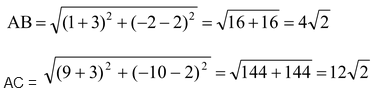Since, AB + BC =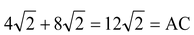, the points A, B and C are collinear.

question 6. Find a point on the X-axis which is equidistant from the points (5, 4) and (-2, 3).

question Since the required point (say P) is on the X-axis, its ordinate will be zero. Let the abscissa of the point be x.

Therefore, coordinates of the point P are (x, 0).

Let A and B denote the points (5, 4) and (–2, 3), respectively.

Since we are given that AP = BP, we have

AP2 = BP2.

i.e., (x – 5)2 + (0 – 4)2 = (x + 2)2 + (0 – 3)2

or x2 + 25 – 10x + 16 = x2 + 4 + 4x + 9

or –14x = –28

or x = 2.

Thus the required point is (2, 0).

question 7. If the distance of P (x, y) from A (5, 1) and B(–1, 5) are equal, prove that 3x = 2y.

question P(x, y), A (5, 1) and B (–1, 5) are the given points.

AP = BP. (Given)

∴ AP2 = BP2

or AP2 – BP2 = 0

or {(x – 5)2 + (y – 1)}2 – {(x + 1)2 + (y – 5)2} = 0

or x2 + 25 – 10x + y2 + 1 – 2y – x2 – 1 – 2x – y2 – 25 + 10y = 0

or –12x + 8y = 0

or 3xx = 2y.